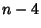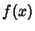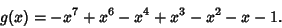## Descartes' Sign Rule

A method of determining the maximum number of Positive and Negative Real Roots of a Polynomial.

For Positive Roots, start with the Sign of the Coefficient of the lowest (or highest) Power. Count the number of Sign changesas you proceed from the lowest to the highest Power (ignoring Powers which do not appear). Thenis the maximum number of Positive Roots. Furthermore, the number of allowable Roots is,,, .... For example, consider the PolynomialSince there are three Sign changes, there are a maximum of three possible Positive Roots.

For Negative Roots, starting with a Polynomial, write a new Polynomialwith the Signs of all Odd Powers reversed, while leaving the Signs of the Even Powers unchanged. Then proceed as before to count the number of Sign changes. Thenis the maximum number of Negative Roots. For example, consider the Polynomialand compute the new PolynomialThere are four Sign changes, so there are a maximum of four Negative Roots.

References

Anderson, B.; Jackson, J.; and Sitharam, M. Descartes' Rule of Signs Revisited.'' Amer. Math. Monthly 105, 447-451, 1998.

Hall, H. S. and Knight, S. R. Higher Algebra: A Sequel to Elementary Algebra for Schools. London: Macmillan, pp. 459-460, 1950.

Struik, D. J. (Ed.). A Source Book in Mathematics 1200-1800. Princeton, NJ: Princeton University Press, pp. 89-93, 1986.